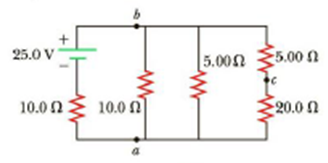Chapter 18, Problem 11P

Chapter
Section
Textbook Problem

Consider the circuit shown in Figure P18.11. Find (a) the potential difference between points a and b and (b) the current in the 20.0-Ω resistor.Figure P18.11

(a)

To determine
The potential difference between the points a and b.

Explanation

Given Info: The bottom of the two resistors connected in series combinations are 20.0Ω and 5.00Ω . The other resistors connected in parallel combinations are 25.0Ω , 10.0Ω and 5.00Ω . The potential difference across the battery is 25.0 V and from the step 3 two resistors is 2.94Ω and 10.0Ω .

Explanation:

Formula to calculate the equivalent resistance of the two resistors connected in series.

Rs=R4+R5

• Rs is the equivalent resistance of the two resistors connected in series,
• R4 and R5 is the resistors,

Substitute 20.0Ω for R4 and 5.00Ω for R5 .

Rs=20.0Ω+5.00Ω=25.0Ω

Thus, the equivalent resistance of the two resistors connected in series is 25.0Ω .

Formula to calculate the equivalent resistance of the resistors connected in parallel.

1Rp=1R2+1R3+1Rs

• Rs is the equivalent resistance of the two resistors connected in series,
• R2 and R3 is the resistors,
• Rp is the equivalent resistance of the resistors connected in parallel,

Substitute 25.0Ω for Rs , 10.0Ω for R2 and 5.00Ω for R3 .

1Rp=110

(b)

To determine
The current passing through the 20.0Ω resistor.

Still sussing out bartleby?

Check out a sample textbook solution.

See a sample solution

The Solution to Your Study Problems

Bartleby provides explanations to thousands of textbook problems written by our experts, many with advanced degrees!

Get Started